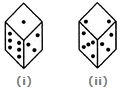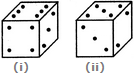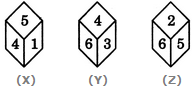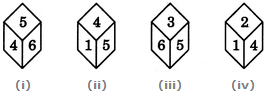# Non Verbal Reasoning - Cubes and Dice

### Exercise :: Cubes and Dice - Section 2

21.

Two positions of a dice are shown below: When 2 is at the bottom, what number will be at the top?A. 6 B. 4 C. 1 D. 5

Answer: Option C

Explanation:

Number 3 is common to both the figures (i) and (ii). The dice in fig. (ii) is assumed to be rotated so that 3 remains on the FR-RH face (i.e. face I as per activity 1) and the numbers 5 and 2 move to the faces hidden behind the numbers 6 and 1 respectively [in fig. (i)]. Thus, the combined figure will have 3 on FR-RH face (i.e. face I), 5 on RR-RH face (i.e. face II), 2 on Bottom face (i.e. face VI), 1 on the Top face (i.e. face V) and 6 on FR-LH face (i.e. face IV). Clearly, 2 lies opposite 1. Hence, when 2 is at the bottom, then 1 will be at the top.

22.

A dice is numbered from 1 to 6 in different ways.

If 1 is opposite to 5 and 2 is opposite to 3, then

 A. 4 is adjacent to 3 and 6 B. 2 is adjacent to 4 and 6 C. 4 is adjacent to 5 and 6 D. 6 is adjacent to 3 and 4

Answer: Option B

Explanation:

If 1 is opposite to 5 and 2 is opposite to 3, then 4 definitely lies opposite to 6. Therefore, 2 cannot lie opposite to any of the two numbers - 4 or 6. Hence, 2 necessarily lies adjacent to both 4 and 6.

23.

Two positions of a block are shown below. When 2 is at the bottom, which number will be at the top?A. 1 B. 4 C. 6 D. Cannot be determined

Answer: Option C

Explanation:

Number 3 is common to the two positions of the block. We assume the block in fig. (ii) to be rotated so that 3 appears at the same position as in fig. (i) i.e. on RHS face (i.e. on face II as per activity 1) and the numbers 5 and 2 move to the faces hidden behind the numbers 4 and 6 respectively [in fig. (i)]. Thus, the combined figure will have 3 on RHS face (i.e. face II), 4 on the Front face (i.e. face I), 6 on the Top face (i.e. face V), 5 on the Rear face (i.e. face III) and 2 on the Bottom face (i.e. face VI). Clearly, when 2 is at the bottom; then 6 is at the top.

24.

Three different positions X, Y and Z of a dice are shown in the figures given below. Which numbers are hidden behind the numbers 6 and 5 in the position Z?A. 1 and 4 B. 1 and 3 C. 4 and 3 D. 1 and 2

Answer: Option B

Explanation:

From positions X and Y we conclude that 1, 5, 6 and 3 lie adjacent to 4. Therefore, 2 must lie opposite 4. From positions Y and Z we conclude that 4, 3, 2 and 5 lie adjacent to 6. Therefore, 1 must lie opposite 6. Thus, 2 lies opposite 4, 1 lies opposite 6 and consequently 5 lies opposite 3.

As analysed above, the number opposite 6 is 1 and the number opposite 5 is 3. Therefore, the numbers hidden behind the numbers 6 and 5 in position Z (these are the numbers opposite 5 and 6 respectively) are 1 and 3.

25.

Four positions of a dice are shown below. What number must be at the bottom face when the dice is in the position as shown in the figure(iii)A. 1 B. 2 C. 4 D. 6

Answer: Option C

Explanation:

From figures (i), (ii), (iv) we conclude that 5, 6, 1 and 2 lie adjacent to 4. Hence, 3 must lie opposite 4 and vice-versa. In fig. (iii), 3 is at the top and consequently 4 must lie at the bottom face.

#### Current Affairs 2021

Interview Questions and Answers